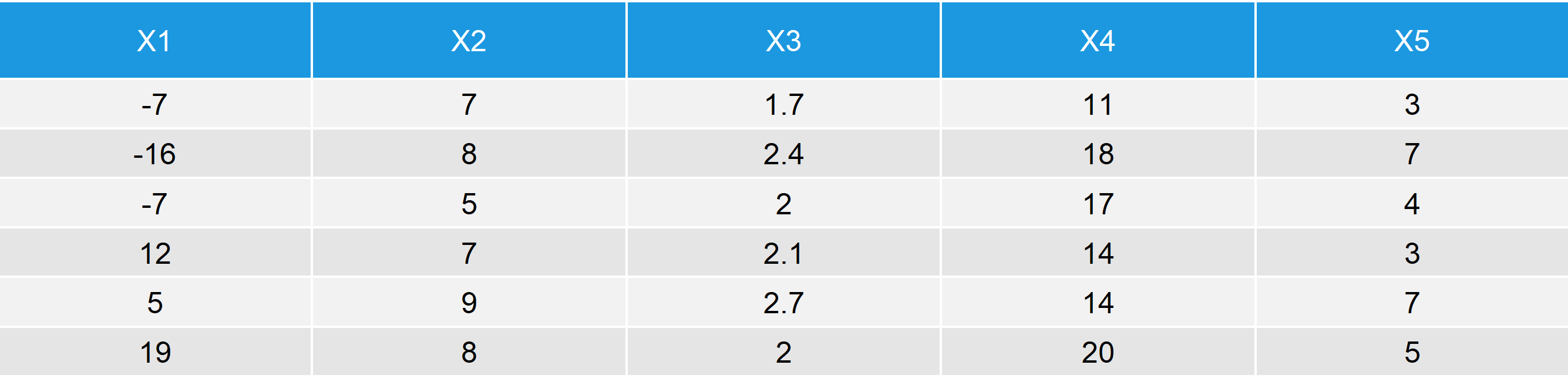# The dim Function in R (4 Examples)

Basic R Syntax:

`dim(data)`

The dim function of the R programming language returns the dimension (e.g. the number of columns and rows) of a matrix, array or data frame. Above, you can see the R code for the application of dim in R.

Continue reading! I’ll provide you with several example codes and practical tips in the following article.

## Example 1: Dimension of Matrix or Data Frame

Let’s first create some example data, before we start with the application of dim in R:

```set.seed(62626)                          # Set Seed for reproducibility
N <- 500                                 # Sample size

x1 <- round(rnorm(N, 0, 10))             # Create 5 random variables
x2 <- round(runif(N, 5, 10))
x3 <- round(runif(N, 1, 3), 1)
x4 <- round(runif(N, 10, 20))
x5 <- rpois(N, 5)

data <- data.frame(x1, x2, x3, x4, x5)   # Data frame with 5 columns
head(data)                               # First 6 rows of data.frame```Table 1: First 6 Rows of Our Example Data Frame for the Application of dim in R.

Table 1 illustrates how our example data.frame looks like. It’s easy to see that the data consists of 5 columns. But how many rows? Let’s check with the dim R function:

```dim(data)                                # Apply dim function to data.frame
# 500 5```

After applying the dim function in R (I use the RStudio interface), we get two numbers back. The first number reflects the number of rows; and the second number reflects the number of columns.

In other words: Our data frame consists of 500 rows and 5 columns.

The same procedure could be applied to a matrix. Let’s convert our data to the matrix format and check if it works:

```data_matrix <- as.matrix(data)           # Convert data.frame to matrix
dim(data_matrix)                         # Apply dim function to matrix
# 500 5```

Same result as before – perfect!

## Example 2 (Video): dim of a Real Data Frame

In the previous example, I have shown you how to apply dim to a synthetic data set. Do you want to see an example that is based on more realistic data? Then you could check out the following video of my YouTube channel Statistical Programming. In the video, I’m showing another example for the application of dim to a data frame:

## Example 3: dim of List in R

Sometimes, it is useful to use dim for a list object in R. That task is easily done with a combination of dim() and sapply().

First, let’s create a list in R:

```data_list <- list()                      # Create empty list object
data_list[] <- data                   # First entry of list
data_list[] <- data[1:10, ]           # Second entry (subset of data)
data_list[] <- data[5:67, c(1, 3, 5)] # Third entry (other subset of data)```

Now, let’s extract the dimensions of each list element:

```sapply(data_list, dim)                   # Get dimension of all list entries
#      [,1] [,2] [,3]
# [1,]  500   10   63
# [2,]    5    5    3```

The combination of dim and sapply returns a matrix to the RStudio console. Each column of this matrix reflects the dimension of one list element:

• List entry 1: 500 rows; 5 columns
• List entry 2: 10 rows; 5 columns
• List entry 3: 63 rows; 3 columns

## Example 4: dim in R Returns NULL – What’s the Problem?

A common mistake is the application of dim to a one dimensional vector or array. Let’s see what happens, when we do this.

I’ll first create an example vector…

`vec1 <- c(5, 9, - 20, 3, 17, 18, 2)      # Example vector`

…and then I’ll apply the dim function:

```dim(vec1)                                # Apply dim function to vector
# NULL```

As you see: That doesn’t work!

In case you want to get the number of entries of a vector, you have to use the length function:

```length(vec1)                             # Get length of vector or array
# 7```